Electron. J. Diff. Eqns., Vol. 2005(2005), No. 57, pp. 1-12.

### A multiplicity result for quasilinear problems with convex and concave nonlinearities and nonlinear boundary conditions in unbounded domains Dimitrios A. Kandilakis

Abstract:
We study the following quasilinear problem with nonlinear boundary conditions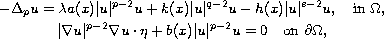where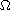is an unbounded domain in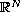with a noncompact and smooth boundary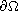,denotes the unit outward normal vector on,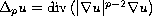is the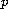-Laplacian,,,andare nonnegative essentially bounded functions,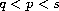and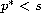. The properties of the first eigenvalue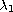and the associated eigenvectors of the related eigenvalue problem are examined. Then it is shown that if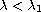, the original problem admits an infinite number of solutions one of which is nonnegative, while if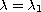it admits at least one nonnegative solution. Our approach is variational in character.

Submitted September 27, 2004. Published May 31, 2005.
Math Subject Classifications: 35J20, 35J60.
Key Words: Variational method; fibering method; Palais-Smale condition; genus.

Show me the PDF file (261K), TEX file, and other files for this article.Dimitrios A. Kandilakis Department of Sciences Technical University Of Crete Chania, Crete, 73100 Greece email: dkan@science.tuc.gr